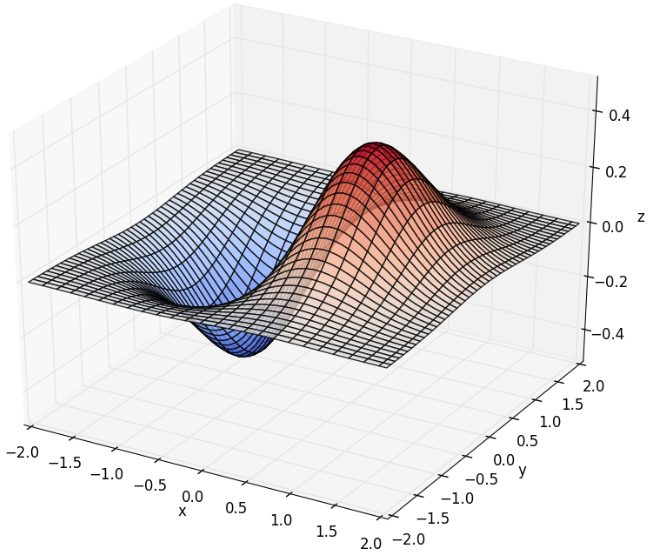## Python数学建模三剑客之Matplotlib

213次阅读matplotlib 是python最著名的绘图库，它提供了一整套和Matlab相似的命令API，十分适合交互式地进行制图。而且也可以方便地将它作为绘图控件，嵌入GUI应用程序中。matplotlib 可以绘制多种形式的图形包括普通的线图，直方图，饼图，散点图以及误差线图等；可以比较方便的定制图形的各种属性比如图线的类型，颜色，粗细，字体的大小等；它能够很好地支持一部分 TeX 排版命令，可以比较美观地显示图形中的数学公式。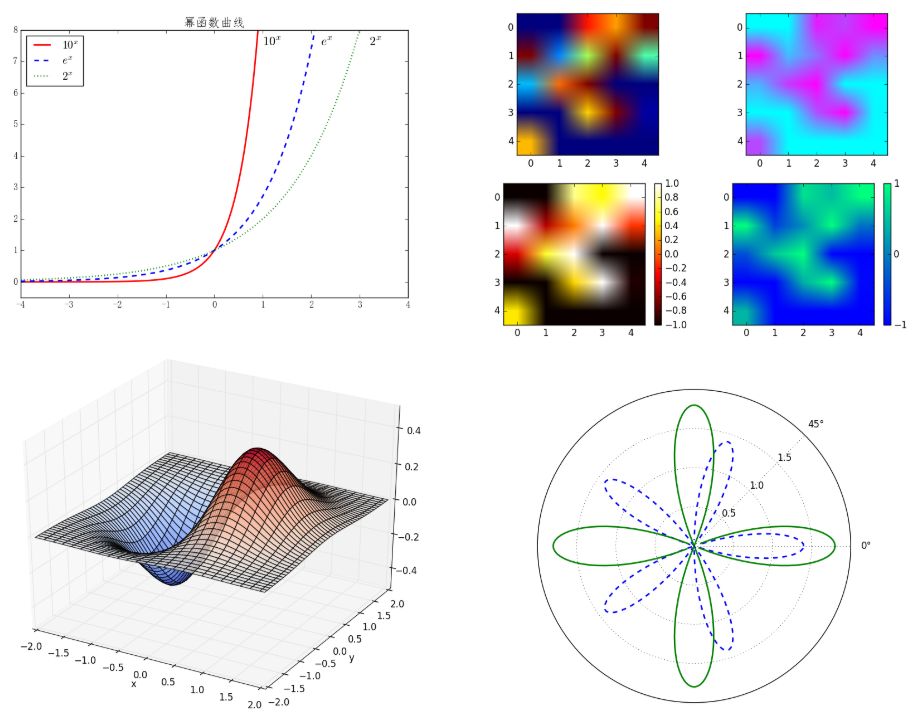3.1 pylot介绍

Matplotlib 包含了几十个不同的模块， 如 matlab、mathtext、finance、dates 等，而 pylot 则是我们最常用的绘图模块，这也是本文介绍的重点。

3.1.1 中文显示问题的解决方案

```>>> import matplotlib.pyplot as plt
>>> plt.rcParams['font.sans-serif'] = ['FangSong'] # 指定默认字体
>>> plt.rcParams['axes.unicode_minus'] = False     # 解决保存图像时'-'显示为方块的问题```

3.1.2 绘制最简单的图形

```>>> import numpy as np
>>> import matplotlib.pyplot as plt
>>> x = np.arange(0, 2*np.pi, 0.01)
>>> y = np.sin(x)
>>> plt.plot(x, y)
>>> plt.show()```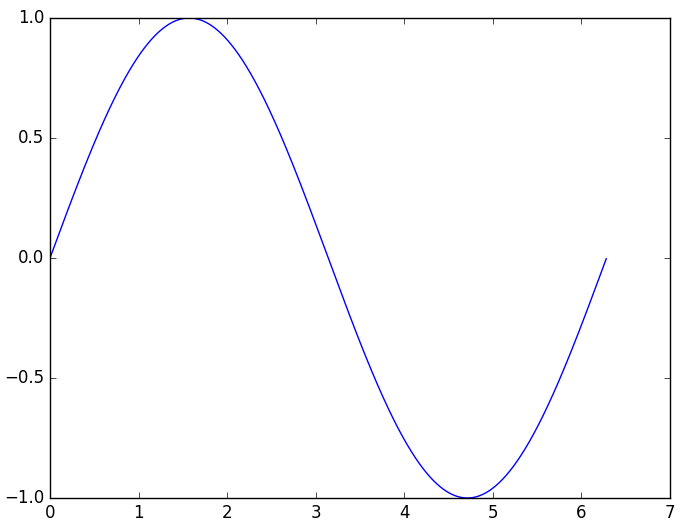3.1.3 设置标题、坐标轴名称、坐标轴范围

```>>> import numpy as np
>>> import matplotlib.pyplot as plt
>>> from pylab import mpl
>>> mpl.rcParams['font.sans-serif'] = ['FangSong']
>>> mpl.rcParams['axes.unicode_minus'] = False
>>> x = np.arange(0, 2*np.pi, 0.01)
>>> y = np.sin(x)
>>> plt.plot(x, y)
>>> plt.title(u'正弦曲线', fontdict={'size':20})      # 设置标题
>>> plt.xlabel(u'弧度', fontdict={'size':16})         # 显示横轴名称
>>> plt.ylabel(u'正弦值', fontdict={'size':16})       # 显示纵轴名称
>>> plt.axis([-0.1*np.pi, 2.1*np.pi, -1.1, 1.1])     # 设置坐标轴范围
>>> plt.axis('equal')                                # xy轴等比例保持
>>> plt.show()```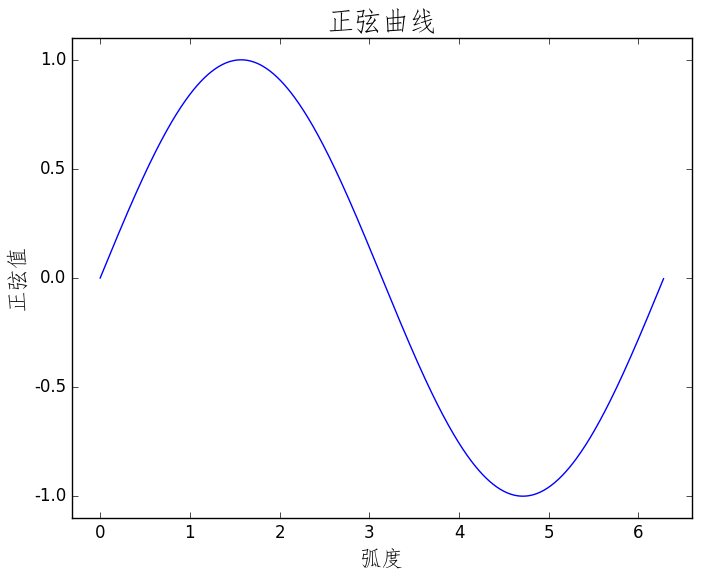3.1.4 设置点和线的样式、宽度、颜色

plt.plot函数的调用形式如下：

```plot(x, y, color='green', linestyle='dashed', linewidth=1, marker='o', markerfacecolor='blue', markersize=6)
plot(x, y, c='g', ls='--', lw=1, marker='o', mfc='blue', ms=6)```

1、color指定线的颜色，可简写为“c”。颜色的选项为：

·蓝色： ‘b’ (blue)

·绿色： ‘g’ (green)

·红色： ‘r’ (red)

·墨绿： ‘c’ (cyan)

·洋红： ‘m’ (magenta)

·黄色： ‘y’ (yellow)

·黑色： ‘k’ (black)

·白色： ‘w’ (white)

·灰度表示： e.g. 0.75 ([0,1]内任意浮点数)

·RGB表示法： e.g. ‘#2F4F4F’ 或 (0.18, 0.31, 0.31)

1、linestyle指定线型，可简写为“ls”。线型的选项为：

·实线： ‘-’ (solid line)

·虚线： ‘–’ (dashed line)

·虚点线： ‘-.’ (dash-dot line)

·点线： ‘:’ (dotted line)

·无： ‘‘或’ ‘或’None’

1、linewidth指定线宽，可简写为“lw”。

2、marker描述数据点的形状

·点线： ‘.’

·点线： ‘o’

·加号： ‘+

·叉号： ‘x’

·上三角： ‘^’

·上三角： ‘v’

1、markerfacecolor指定数据点标记的表面颜色，可 简写为“ mfc”。

2、markersize指定数据点标记的大小，可 简写为“ ms”。

3.1.5 文本标注和图例

```>>> import numpy as np
>>> import matplotlib.pyplot as plt
>>> from pylab import mpl
>>> mpl.rcParams['font.sans-serif'] = ['FangSong']
>>> mpl.rcParams['axes.unicode_minus'] = False
>>> x = np.linspace(-4, 4, 200)
>>> f1 = np.power(10, x)
>>> f2 = np.power(np.e, x)
>>> f3 = np.power(2, x)
>>> plt.plot(x, f1, 'r', ls='-', linewidth=2, label='\$10^x\$')
>>> plt.plot(x, f2, 'b', ls='--', linewidth=2, label='\$e^x\$')
>>> plt.plot(x, f3, 'g', ls=':', linewidth=2, label='\$2^x\$')
>>> plt.axis([-4, 4, -0.5, 8])
>>> plt.text(1, 7.5, r'\$10^x\$', fontsize=16)
>>> plt.text(2.2, 7.5, r'\$e^x\$', fontsize=16)
>>> plt.text(3.2, 7.5, r'\$2^x\$', fontsize=16)
>>> plt.title('幂函数曲线', fontsize=16)
>>> plt.legend(loc='upper left')
>>> plt.show()```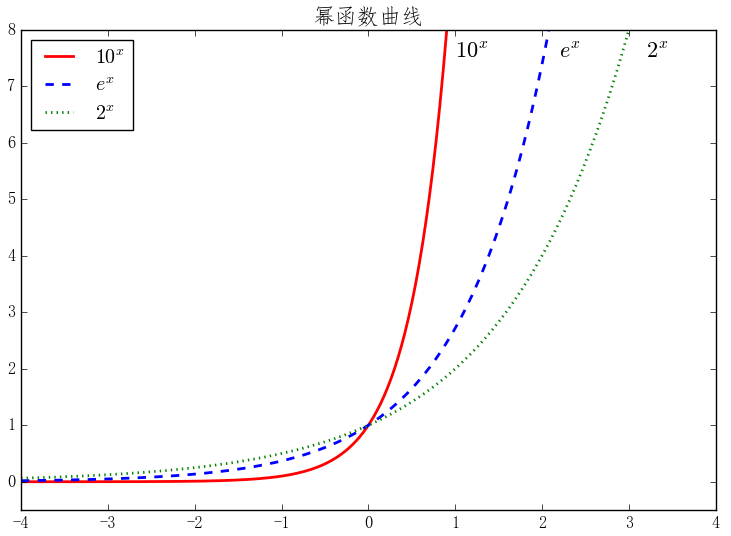·best

·upper right

·upper left

·lower left

·lower right

3.2 绘制多轴图

`subplot(numRows, numCols, plotNum)`

subplot将整个绘图区域等分为numRows行 * numCols列个子区域，然后按照从左到右，从上到下的顺序对每个子区域进行编号，左上的子区域的编号为1。如果numRows，numCols和plotNum这三个数都小于10的话，可以把它们缩写为一个整数，例如subplot(323)和subplot(3,2,3)是相同的。subplot在plotNum指定的区域中创建一个轴对象。如果新创建的轴和之前创建的轴重叠的话，之前的轴将被删除。

```>>> import matplotlib.pyplot as plt
>>> plt.subplot(221) # 两行两列的第1个位置
>>> plt.axis([-1, 2, -1, 2])
>>> plt.axhline(y=0.5, color='b')
>>> plt.axhline(y=0.5, xmin=0.25, xmax=0.75, color='r')
>>> plt.subplot(222) # 两行两列的第2个位置
>>> plt.axis([-1, 2, -1, 2])
>>> plt.axvline(x=0, ymin=0, linewidth=4, color='r')
>>> plt.axvline(x=1.0, ymin=-0.5, ymax=0.5, linewidth=4, color='g')
>>> plt.subplot(212) # 两行一列的第2个位置
>>> plt.axis([-1, 2, -1, 2])
>>> plt.axvspan(1.25, 1.55, facecolor='g', alpha=0.5)
>>> plt.axhspan(0.25, 0.75, facecolor='0.5', alpha=0.5)
>>> plt.show()```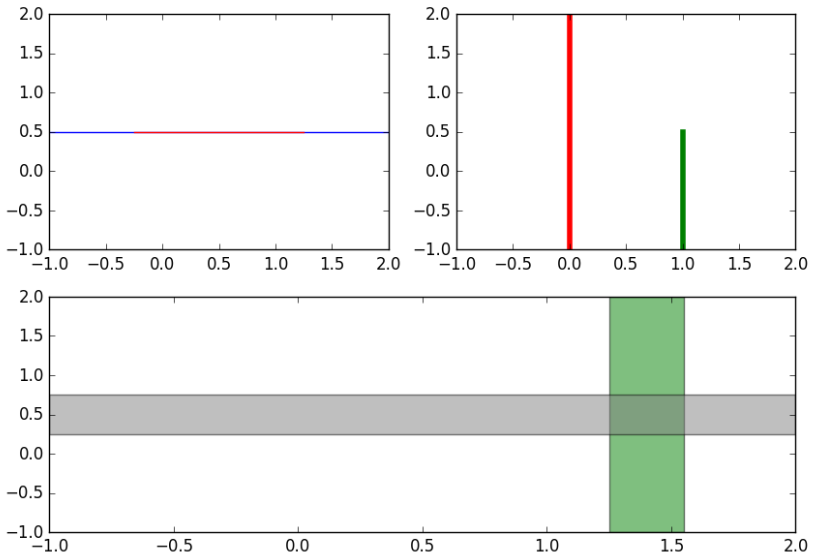3.3 常用绘图类型

3.3.1 直方图

```>>> import numpy as np
>>> import matplotlib.pyplot as plt
>>> data = np.random.normal(5.0, 3.0, 1000)
>>> plt.hist(data)
>>> bins = np.arange(-5, 16, 1)
>>> plt.hist(data, bins) # 使用自定义的分段区域
>>> plt.show()```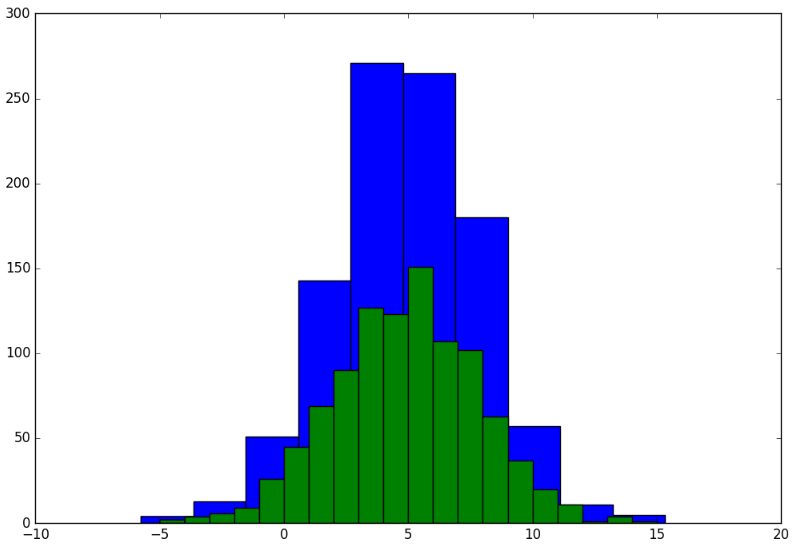3.3.2 散点图

plt.scatter函数的调用形式如下：

```scatter(x,y,s=None,c=None,marker=None,cmap=None,norm=None,vmin=None,vmax=None,alpha=None,linewidths=None,verts=None,
edgecolors=None,hold=None,data=None,**kwargs)```

scatter()的前两个参数是数组，分别指定每个点的X轴和Y轴的坐标。s参数指定点的大 小，值和点的面积成正比，它可以是一个数，指定所有点的大小，也可以是数组，分别对每个点指定大小。c参数指定每个点的颜色，可以是数值或数组。这里使用一维数组为每个点指定了一个数值。通过颜色映射表，每个数值都会与一个颜色相对应。默认的颜色映射表中蓝色与最小值对应，红色与最大值对应。当c参数是形状为(N,3)或(N,4)的二维数组时，则直接表示每个点的RGB颜色。marker参数设置点的形状，可以是个表示形状的字符串，也可以是表示多边形的两个元素的元组，第一个元素表示多边形的边数，第二个元素表示多边形的样式，取值范围为0、1、2、3。0表示多边形，1表示星形，2表示放射形，3表示忽略边数而显示为圆形。alpha参数设置点的透明度。facecolors参数为“none”时，表示散列点没有填充色。

```>>> import numpy as np
>>> import matplotlib.pyplot as plt
>>> x = np.random.rand(50)
>>> y = np.random.rand(50)
>>> area = np.pi * (15 * np.random.rand(50))**2
>>> color = 2 * np.pi * np.random.rand(50)
>>> plt.scatter(x, y, s=area, c=color, alpha=0.5, cmap=plt.cm.hsv)
>>> plt.show()```3.3.3 梯形图、柱状图、填充图

```>>> import numpy as np
>>> import matplotlib.pyplot as plt
>>> n = np.array([0,1,2,3,4,5])
>>> x = np.linspace(-0.75, 1., 100)
>>> plt.subplot(131)
>>> plt.step(n, n**2, lw=2)
>>> plt.subplot(132)
>>> plt.bar(n, n**2, align="center", width=0.5, alpha=0.5)
>>> plt.subplot(133)
>>> plt.fill_between(x, x**2, x**3, color="green", alpha=0.5)
>>> plt.show()```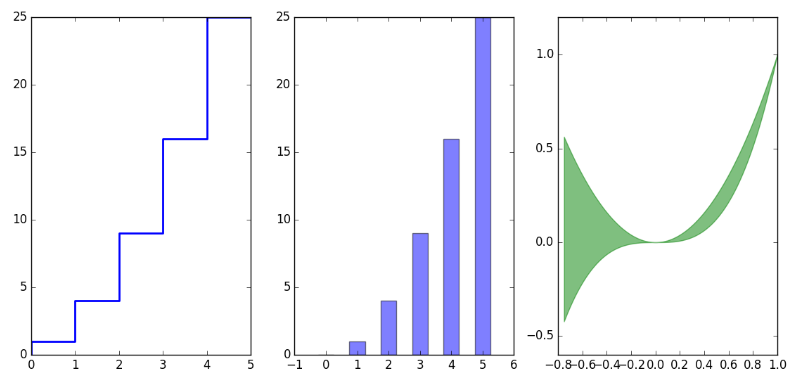3.3.4 对数坐标

plot()所绘制图表的X-Y轴坐标都是算术坐标。绘制对数坐标图的函数有三个：semilogx()、semilogy()和loglog(),它们分别绘制X轴为对数坐标、Y轴为对数坐标以及两个轴都为对数坐标时的图表。

```>>> import numpy as np
>>> import matplotlib.pyplot as plt
>>> x = np.linspace(0, 3, 100)
>>> y = np.power(2, np.power(2,x))
>>> plt.subplot(121)
>>> plt.semilogy(x, y , '-r')
>>> plt.subplot(122)
>>> plt.plot(x,y, '--g')
>>> plt.show()```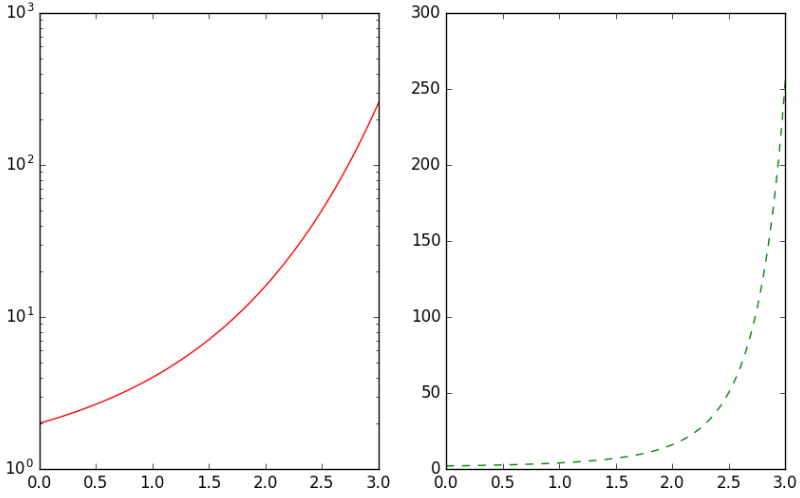3.3.5极坐标绘图

```>>> import numpy as np
>>> import matplotlib.pyplot as plt
>>> theta = np.arange(0, 2*np.pi, 0.02)
>>> plt.polar(theta, 1.4*np.cos(5*theta), "--", linewidth=2)
>>> plt.polar(theta, 1.8*np.cos(4*theta), linewidth=2)
>>> plt.rgrids(np.arange(0.5, 2, 0.5), angle=45)
>>> plt.thetagrids([0, 45])thetagridlabel objects>)
>>> plt.show()
>>>```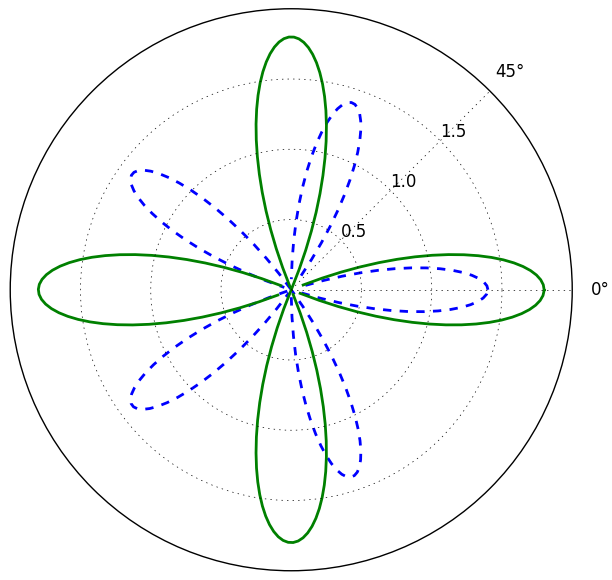3.4 2D绘图

3.4.1 等值线图

```>>> import numpy as np
>>> import matplotlib.pyplot as plt
>>> y, x = np.ogrid[-2:2:200j, -3:3:300j]
>>> z = x * np.exp( - x**2 - y**2)
>>> extent = [np.min(x), np.max(x), np.min(y), np.max(y)]
>>> plt.subplot(121)
>>> cs = plt.contour(z, 10, extent=extent)
>>> plt.clabel(cs)
<a list of 8 text.Text objects>
>>> plt.subplot(122)
>>> plt.contourf(x.reshape(-1), y.reshape(-1), z, 20)
>>> plt.show()```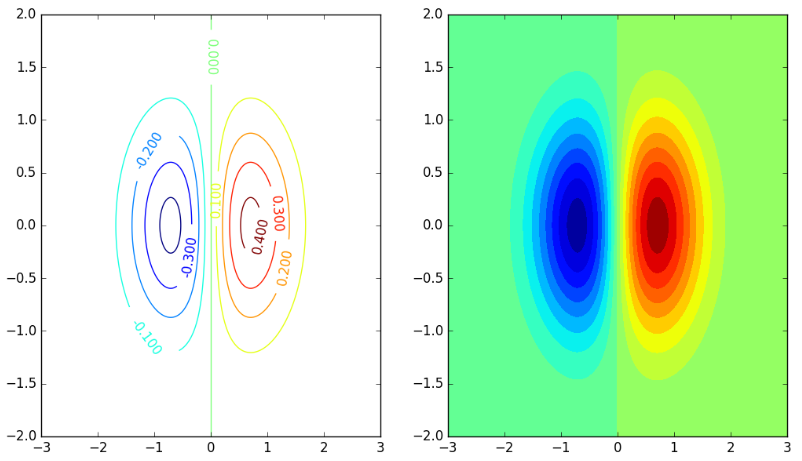3.4.2 二维数据的平面色彩显示

```>>> import numpy as np
>>> import matplotlib.pyplot as plt
>>> data=np.clip(np.random.randn(5,5),-1,1)
>>> plt.subplot(221)
>>> plt.imshow(data)
>>> plt.subplot(222)
>>> plt.imshow(data,cmap=plt.cm.cool)
>>> plt.subplot(223)
>>> plt.imshow(data,cmap=plt.cm.hot)
>>> plt.colorbar()
>>> plt.subplot(224)
>>> im = plt.imshow(data,cmap=plt.cm.winter)
>>> plt.colorbar(im, cmap=plt.cm.winter, ticks=[-1,0,1])
>>> plt.show()```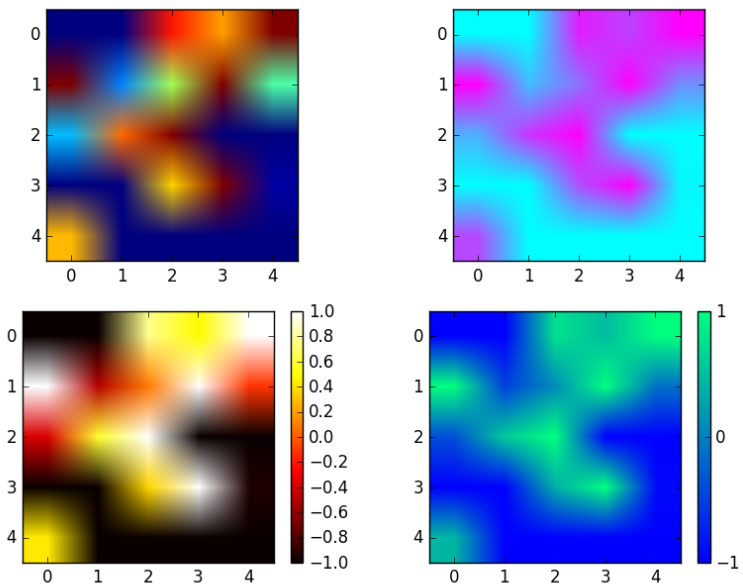3.5 3D绘图

mpl_toolkits.mplot3工具包提供了一些基本的3D绘图功能，其支持的图表类型包括散点图（scatter）、曲面图（surf）、线图（line）和网格图（mesh）。虽然mplot3d不是一个最好的3D图形绘制库，但是它是伴随着matplotlib产生的，因此我们对其接口已经很熟悉了。

```>>> import numpy as np
>>> import matplotlib.pyplot as plt
>>> import mpl_toolkits.mplot3d
>>> x, y = np.mgrid[-2:2:50j,-2:2:50j]
>>> z = x*np.exp(-x**2-y**2)
>>> ax = plt.subplot(111,projection='3d')
>>> ax.plot_surface(x,y,z,rstride=2,cstride=1,cmap=plt.cm.coolwarm,alpha=0.8)
>>> ax.set_xlabel('x')
>>> ax.set_ylabel('y')
>>> ax.set_zlabel('z')
>>> plt.show()```